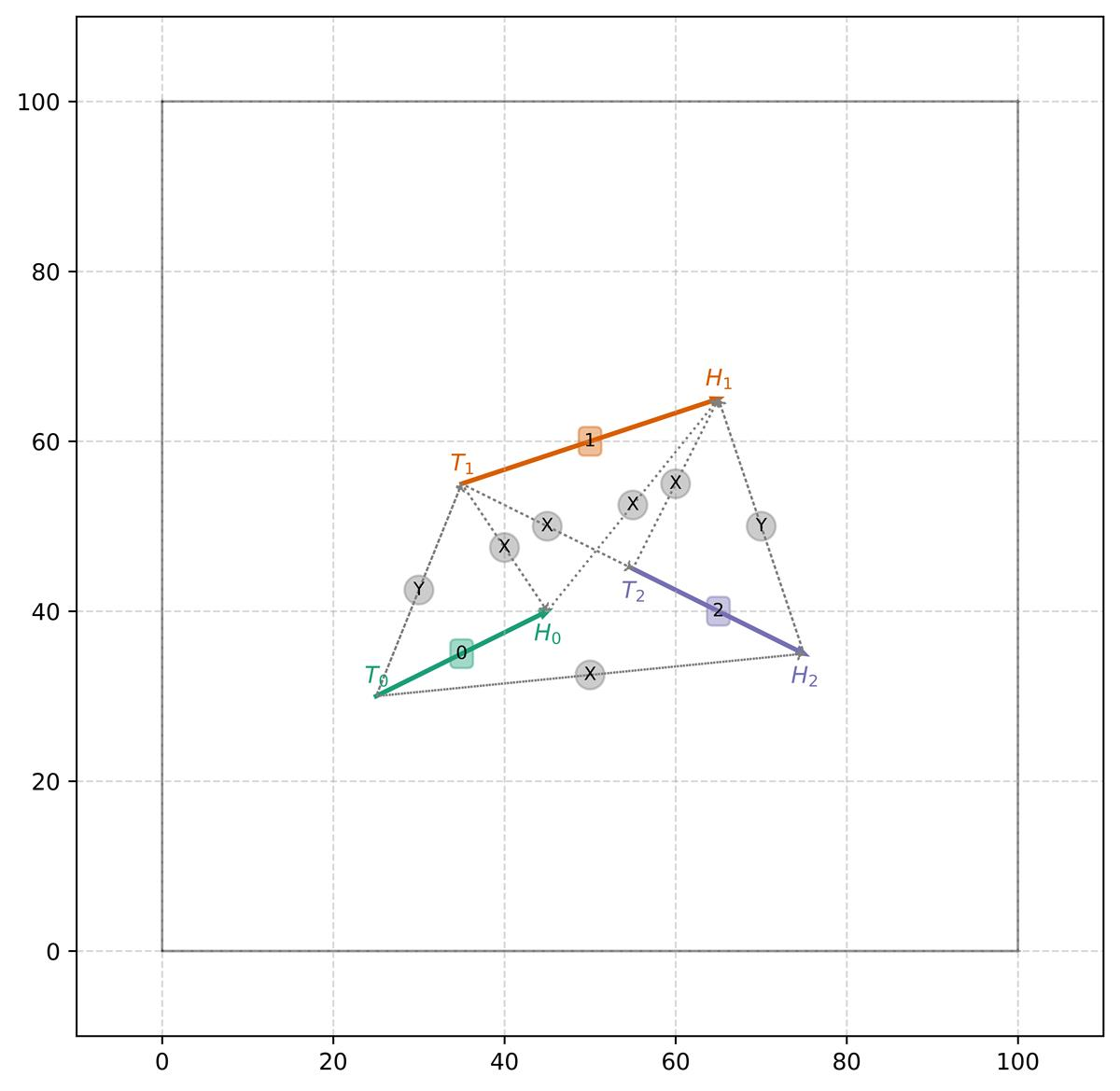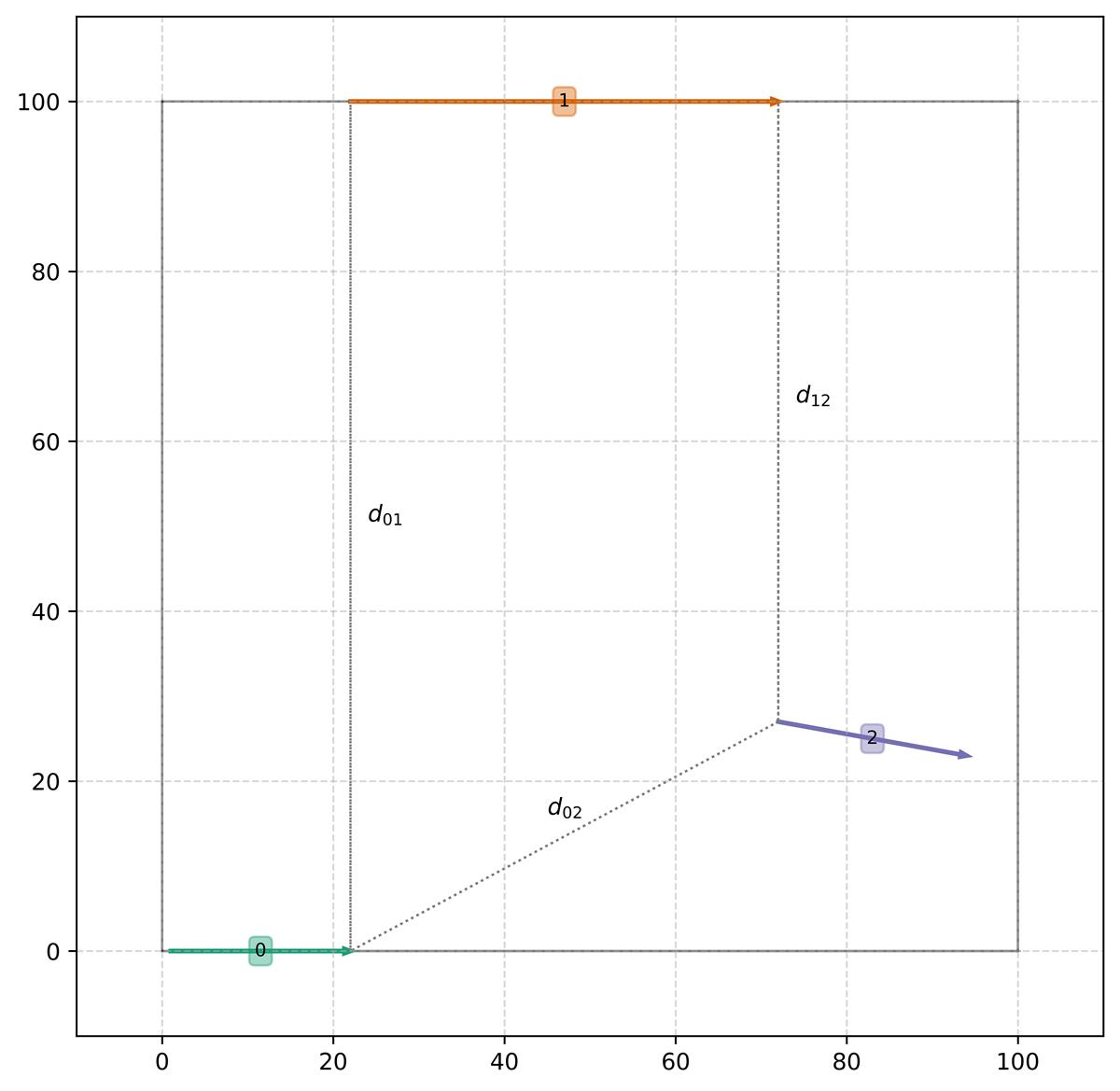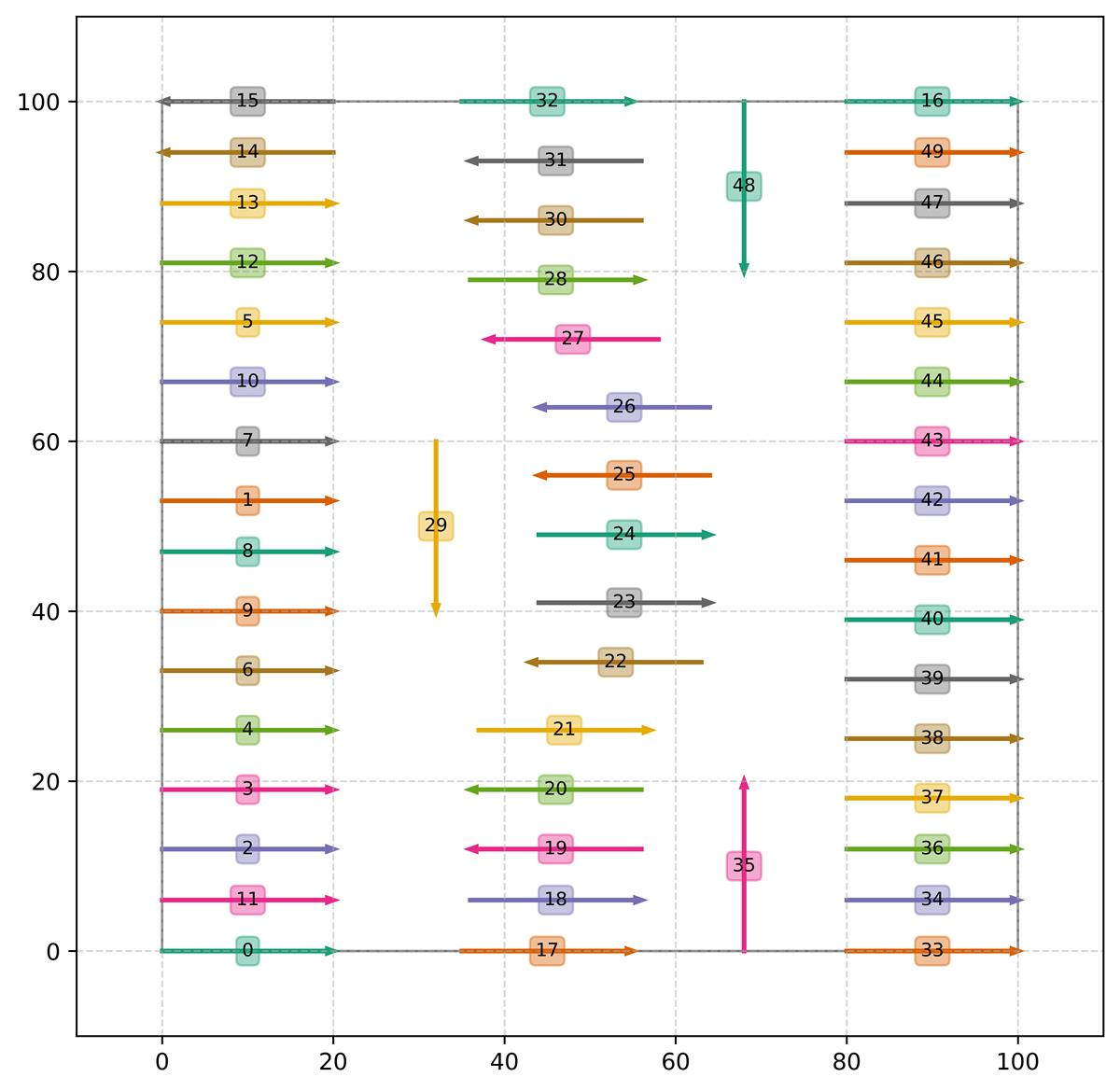View Only

# Black-box expressions

Constraint Programming (CP) is known for its expressiveness for modeling and solving combinatorial optimization problems. In particular, CP uses predefined expressions, such as product or division between variables (`x*y*z`, `x/y`), logarithm (`log(x)`), power (`x^y`), etc. that go beyond classical linear or quadratic expressions used in Mathematical Programming. Still, there are cases when this is not enough and we would like to model some numerical quantities that cannot be expressed as a closed-form expression. Typical examples include:

• Legacy code where one has no access to what is inside a library/executable
• Numerical code involving differential equations, integrals, or any computation that cannot be easily expressed using predefined CP Optimizer expressions
• Result of a complex simulation
• Prediction of a machine learning model
• Real-life experiments: crash tests, chemical reactions, etc.
• Result of an interaction with a user

CP Optimizer 20.1 introduces the concept of black-box expression in the model. Black-box expressions are known in black-box optimization and in local search solvers. They are much less common in exact solvers like the ones using CP technologies.

A black-box expression is specified by giving a (C++) function that evaluates the expression at given points.

Blackbox expressions are defined using macros `ILOBLACKBOXn` where n is the number of arguments of the black-box:

`  ILOBLACKBOXn(name, type1, arg1, type2, arg2, ..., typen, argn) { EXPRESSION EVALUATION CODE }`

Example:

`  ILOBLACKBOX2(f, IloIntVar, x, IloIntVar, y) { EXPRESSION EVALUATION CODE }`

Will define a black-box expression f(x,y) taking as argument two integer variables whose signature in the model is :

`  IloNumExpr f(IloEnv env, IloIntVar x, IloIntVar y);`

## Scope

The CP Optimizer engine can call a user-defined black-box evaluation function as soon as all the variables and expressions in its scope are fixed.

The scope of the black-box expression is the set of CP Optimizer variables or expressions which, when fixed during solution search, will trigger a call to the black-box function. When you pass any of the following types to the `ILOBLACKBOX` macros, they will be recognized as belonging to the scope of the expression:

• Integer variables:` IloIntVar`and `IloIntVarArray`
• Integer expressions: `IloIntExpr` and `IloIntExprArray`
• Numerical expressions: `IloNumExpr` and `IloNumExprArray`
• Interval variables: `IloIntervalVar` and `IloIntervalVarArray`
• Sequence variables: `IloIntervalSequenceVar` and `IloIntervalSequenceVarArray`

Additionally, any variable belonging to an n-dimensional array defined by macro `IloArray` on the above types is also automatically recognized as belonging to the scope of the expression. For instance `IloArray<IloArray<IloIntExprArray>>`.

Note that other types of arguments can be passed to the black-box macro like any user-defined structure that can be useful for evaluating the function. These non-recognized arguments will not be considered as being part of the scope of the expression.

Example: The black-box expression below defines an expression with user-defined structure struct and with scope x (array of numerical expressions) and y (array of interval variables).

`  ILOBLACKBOX3(f, MyStructure*, object, IloNumExprArray, x, IloIntervalVarArray, y) {    EXPRESSION EVALUATION CODE   }`

## Use of black-box expressions in the model

A black-box expression can be used in the same way as classical numerical expressions in the model. In particular it can be:

• Mixed with other expressions (classical or black-box expressions):
• `f(env,x,y)*IloLog(x)`
• Used in or as part of the objective function:
• `IloMinimize(env, f(env,x,y))`
• `IloMinimize(env, IloStaticLex(env, f(env,x,y)*IloLog(x), x*y))`
• Used in constraints:
• `model.add(x+y<=f(env,x,y))`
• `model.add(f(env,x,y)==1)`
• Used in the scope of other black-box expressions:
• `f(env, g(env,x,y), 2*IloLog(f(env,x,z)))`
• Used as KPI or part of a KPI:
• `cp.addKPI(IloLog(f(env,x,y)), "logf")`

Using black-box expressions does not impact the completeness of the search: the CP Optimizer search is an exact algorithm that provides optimality proofs. Of course, the use of black-box expressions may make it harder to prove optimality so for instance a good idea can be, if a particular black-box expression `f(X)` is part of the minimization objective function to add some lower bounding constraints `LB(X)≤f(X)` where `LB(X)` is a closed form expression. This is a typical use-case of mixing black-box and classical expression in the model.

## Principal functions accessible in expression evaluation code

### Accessors

The following functions are available to access the current value of the variables/expressions in the scope of the black-box:

`  IloInt  getValue   (IloIntVar x)      const;`
`  IloInt  getValue   (IloIntExpr x)     const;`
`  IloNum  getValue   (IloNumExpr x)     const;`
`  IloBool isPresent  (IloIntervalVar x) const;`
`  IloBool isAbsent   (IloIntervalVar x) const;`
`  IloInt  getStart   (IloIntervalVar x) const;`
`  IloInt  getEnd     (IloIntervalVar x) const;`
`  IloInt  getSize    (IloIntervalVar x) const;`

`  IloIntervalVar getFirst(IloIntervalSequenceVar s) const;`
`  IloIntervalVar getLast (IloIntervalSequenceVar s) const;`
`  IloIntervalVar getNext (IloIntervalSequenceVar s, IloIntervalVar x) const;`
`  IloIntervalVar getPrev (IloIntervalSequenceVar s, IloIntervalVar x) const;`

### Return value

The computed return value can be returned by calling:

`  void returnValue(IloNum val);`

Example: Here is a full example of black-box expression definition:

`  IloNum PI = 3.14159265358979323846;`

`  ILOBLACKBOX2(f, IloNumExpr, x, IloNumExpr, y) {`
`    // f(x,y) = cos4πx + cos4πy + 5(x+y) + 2`
`    IloNum vx = getValue(x);`
`    IloNum vy = getValue(y);`
`    IloNum v = cos(4*PI*vx) + cos(4*PI*vy) + 5*(vx+vy) + 2;`
`    returnValue(v);`
`  }`

### Undefined points

Black-box expressions do not need to be defined on the complete Cartesian product of their variables domain. If the expression is undefined for a particular combination of values, the evaluation code may just omit to return any value. Implicitly this means that this combination of values is not allowed in any feasible solution.

Example:
`  ILOBLACKBOX2(g, IloIntVar, x, IloIntVar, y) {`
`    // g(x,y) = sqrt( (x+y-5)/(x-y) )`
`    IloNum vx = getValue(x);`
`    IloNum vy = getValue(y);`
`    if (vx!=vy) {`
`      IloNum u = (vx+vy-5)/(vx-vy);`
`      if (0<=u) {`
`        returnValue(sqrt(u));`
`      }`
`    }`
`    // Outside of the definition domain of g(x,y) no value is returned`
`  }`

### Returning multiple values

It is sometimes useful for the black-box function to return a vector of values rather than a single value.

Example: Consider a black-box function that performs some simulation using uncertain data, it may be interesting to return different descriptive statistics (like mean, standard deviation, etc.) computed in the same evaluation of the black-box to be used in the model formulation.

The following function is available in the black-box evaluation code to return the i-th component of the returned vector:

`  void returnValue(IloInt i, IloNum val);`

Example: Here is a template of a black-box that would compute some descriptive statistics (mean, standard deviation) as a pair after performing a simulation based on the decision variables x:

`  #define AVRGE 0`
`  #define STDEV 1`

`  ILOBLACKBOX1(simulation, IloIntVarArray, x) {`
`    IloNum avrge, stdev;`
`    // Compute descriptive statistics based on values of decision variables getValue(x[i])`
`    // ...`
`    returnValue(AVRGE, avrge);`
`    returnValue(STDEV, stdev);`
`  }`
The object returned when creating a black-box is in fact an object, subclass of `IloBlackBox` that is more complex than just a numerical expression (`IloNumExpr`). It can be thought of as an array of such expressions whose size is the size of the returned vector of values.
Individual expressions can be retrieved by using the `operator[]` on the concert black-box object.

So, in the model formulation, one can write:
`  IloIntVarArray x(env);`
`  // ...`
`  IloBlackbox stats = simulation(env,x);`
`  IloNumExpr avrge = stats[AVRGE];`
`  IloNumExpr stdev = stats[STDEV];`

By default, when a single value is returned, the black-box can transparently be thought of as a single numerical expression:
There is an automatic cast from an `IloBlackbox f` to an `IloNumExpr` as` f`
In black-box evaluation code, function `returnValue(val)` is just a shortcut for` returnValue(0,val)`

## Other useful functions accessible in expression evaluation code

#### Current expression bounds

The current bounds on the returned (i-th) black-box expression are accessible from within the black-box evaluation code using:

`  IloNum getLB(IloInt i =0);`
`  IloNum getUB(IloInt i =0);`

These functions return values such that, if the value of the black-box expression is outside these bounds, at least one constraint in the model is violated. They can therefore be used to speed-up the evaluation for instance if it becomes clear that the computed value will fall out of these bounds.

#### Parallelism and critical sections

The code evaluating a black-box expression is called by the different parallel workers solving the problem. In case the computation has some side-effect, for instance on a user-defined structure (global structure, or a structure passed as argument to the macro), it may be useful to protect some part of the code from concurrent access by the workers. Critical sections of the evaluation code can be protected using the following functions:

`  void lock();`
`  void unlock();`

#### Other functions

Some facilities are available from within the evaluation function (local random generator, local memory allocator) to make it easier to write multi-thread safe code.

# Illustrative example

We give below a complete example to illustrate the use of black-box expressions in CP Optimizer.

Note that a more involved example is available with CPLEX Studio 20.1 that deals with a job-shop scheduling problem with uncertain activity durations. In that example, a black-box expression is used to perform some simulation on the sequence of operations on the machines in order to estimate the value of the objective function which is the average makespan. You can find this example in `CPLEX_Studio_201/cpoptimizer/examples/src/cpp/sched_jobshop_blackbox.cpp`.

## Problem formulation

Let’s illustrate the use of black-box expressions on an example. Suppose we want to position n vectors V0, …, Vi, … Vn-1 in the Euclidean plane. Each vector Vi joins two points Ti (tail of Vi) and Hi (head of vector Vi).  The x and y coordinates of a point P are respectively denoted x(P) and y(P). The length of vector Vi is denoted li.

We consider three types of constraints in the problem:

• The heads and tails of the vectors can be constrained to belong to some sub-rectangles
• The length of each vector is constrained to belong to a range of values [LMini,LMaxi]
• There are some constraints on the relative positions of vectors given as linear inequalities x(Pi)≤x(Pj) or y(Pi)≤y(Pj) where Pand Pj denotes either the heads or tails of two vectors Vand Vj. For instance a constraint y(Tj)≤y(Hi) states that vector Vi must end (y(Hi)) higher (on the y-axis) than the start of vector Vj (y(Tj)).

A instance with 3 vectors V0, V1, V2 is depicted in the figure below where each vector is colored and gray arcs are relative positioning constraints that connect two vectors' endpoints (the label x or y on the arc denotes on which axis the constraints is posted).

• ∀ i: 0 ≤ x(Ti) ≤ 100, 0 ≤ x(Hi) ≤ 100
• 20 ≤ l0 ≤ 25, 20 ≤ l1 ≤ 50, 20 ≤ l2 ≤ 25,
• y(T0)≤y(T1),  y(H2)≤y(H1),  x(T1)≤x(H0), x(T1)≤x(T2), x(H0)≤x(H1), x(T2)≤x(H1), x(T0)≤x(H2)Finding a feasible solution to a general version of this problem is NP-Complete. The constraints of this problem can easily be expressed in CP Optimizer using integer variables to represent the coordinates of the heads and tails of the vectors and simple predefined constraints for constraining vector length and relative positions.

Now, we are interested in an optimization version of this problem where the objective function to minimize is some expression on the global "interaction" between the vectors. Let dij denote the "shortest distance" between two vectors Vi and Vj (see the figure below). The interaction between two vectors Vi and Vj is defined as 1/ (1+dij2). The objective of the problem is to position the n line vectors while respecting the length and positioning constraints and minimizing the total interaction between pairs of vectors.An example of an optimal solution for this small instance is shown below:

##CP Optimizer model

Here is the complete C++ code for this model.

We will use a black-box expression to compute the distance between two vectors. In fact, this expression could be formulated in a closed form suing CP Optimizer but it would be a bit complex and heavy. Also, the advantage of the black-box approach is that it easily generalizes to more complex shapes or more complex interaction functions that would not allow for a closed form expression.

We suppose we are given a function that computes the distance between two line segments [(x11,y11),(x12,y12)] and [(x21,y21),(x22,y22)] in the Euclidian plane:

`double segments_distance(double x11, double y11, double x12, double y12,`
`                         double x21, double y21, double x22, double y22);`

The following definitions are used for representing points and vectors:

`typedef IloIntVarArray Point;    // P: [X,Y]  A point is two integer variables`
`typedef IloArray<Point> Vector;  // V: [T,H]  A vector is two points`

`#define X 0`
`#define Y 1`
`#define T 0`
`#define H 1`

We define a black-box expression for computing the square of the distance between two vectors a and b:

`ILOBLACKBOX2(SquareDistance, Vector, a, Vector, b) {`
`  IloNum x11 = getValue(a[T][X]);`
`  IloNum y11 = getValue(a[T][Y]);`
`  IloNum x12 = getValue(a[H][X]);`
`  IloNum y12 = getValue(a[H][Y]);`
`  IloNum x21 = getValue(b[T][X]);`
`  IloNum y21 = getValue(b[T][Y]);`
`  IloNum x22 = getValue(b[H][X]);`
`  IloNum y22 = getValue(b[H][Y]);`
`  IloNum d = segments_distance(x11,y11,x12,y12,x21,y21,x22,y22);`
`  returnValue(d*d);`
`}`

Now, we are ready to formulate the problem by mixing predefined CP Optimizer constraints and expressions and the above black-box expression.

Suppose that we have n vectors in the problem, that the minimal and maximal length is given by arrays LMin[i] and LMax[i] for each vector i and the minimal and maximal values for the x and y coordinates of the endpoints are similarly given by a set of arrays TXMin[i], TXMax[i], ... Decision variables of the problem can be created this way, together with the constraints on vector length:

`IloEnv env;`
`IloModel model(env);`
`IloArray<Vector> vec(env,n);`
`for (IloInt i=0; i<n; ++i) {`
`  vec[i] = Vector(env, 2);`
`  vec[i][T] = Point(env, 2);`
`  vec[i][H] = Point(env, 2);`
`  vec[i][T][X] = IloIntVar(env, TXMin[i], TXMax[i]);`
`  vec[i][T][Y] = IloIntVar(env, TYMin[i], TYMax[i]);`
`  vec[i][H][X] = IloIntVar(env, HXMin[i], HXMax[i]);`
`  vec[i][H][Y] = IloIntVar(env, HYMin[i], HYMax[i]);`
`  IloNumExpr l2 = IloSquare(vec[i][T][X]-vec[i][H][X]) +`
`                  IloSquare(vec[i][T][Y]-vec[i][H][Y]);`
`  model.add(IloRange(env, LMin[i]*LMin[i], l2, LMax[i]*LMax[i])); // Min/max vector length`
`}`If there are c relative positioning constraints with their characteristics stored as a tuple array CT so that a constraint ct in this array is described by:

• ct=0 if the constraint is on the x-axis, ct=1 if it is on the y-axis
• ct is the index of the first vector of the constraint
• ct=0 if the constraint holds on the tail of the first vector, ct=1 if it holds on the head
• ct is the index of the second vector of the constraint
• ct=0 if the constraint holds on the tail of the second vector, ct=1 if it holds on the head

The relative positioning constraints can stated as follows:

`for (IloInt k=0; k<c; ++k) {`
`  auto ct = CT[k];`
`  model.add( vec[ct][ct][ct] <= vec[ct][ct][ct] );`
`}`

The objective function to minimize is the sum of the pairwise interaction of the vectors:

`IloNumExpr obj(env);`
`for (IloInt i=0; i<n; ++i) {`
`  for (IloInt j=i+1; j<n; ++j) {`
`    IloNumExpr d2_ij = SquareDistance(env, vec[i], vec[j]);`
`    obj += (1.0 / (1.0 + d2_ij));`
`    model.add(0 <= d2_ij);`
`  }`
`}`
`model.add(IloMinimize(env, obj));`

And ... that's all.

This formulation creates a quadratic number of black-box expressions. An alternative formulation could have been to create a single black-box expression with all the vectors in its scope that directly computes the objective value. An advantage of the presented model is that it can evaluate the small black-box expressions on the fly during the search as soon as two vectors' endpoints are fixed so that it can better exploit engine inferences.

Solving the problem is just a matter of creating an instance of the engine and calling the automatic solve, setting some search parameters if needed:

`IloCP cp(model);`
`cp.solve();`

Here is for instance a solution for a larger problem with 50 equivalent vectors of length between 20 and 25 in a 100x100 square without any relative position constraints and with objective value 2.219286.#cpoptimizer#CPLEX

​​